Time: 3 Hours                                                                                                     Max. Marks: 100

NOTE: There are 9 Questions in all.

·      Question 1 is compulsory and carries 20 marks. Answer to Q. 1. must be written in the space provided for it in the answer book supplied and nowhere else.

·      Out of the remaining EIGHT Questions answer any FIVE Questions. Each question carries 16 marks.

·      Any required data not explicitly given, may be suitably assumed and stated.

Q.1       Choose the correct or best alternative in the following:                                         (2x10)

a.       How many layers are there in the Internet Reference Model?

(A)    3                                                  (B)  4

(C)  5                                                  (D)  7

b.      The IP address with all 0’s represents

(B)    loopback address                         (B)  this computer

c.   In which of the following protocols, the checksum field in the header is optional?

(A)    TCP                                             (B)  IP

(C)  UDP                                            (D)  None of the above.

d.   Using FTP, file can be transferred

(A)    in either direction.

(B)    from local computer to remote only.

(C)  from remote computer to local only.

(D)  none of the above.

e.   Which of the following is not a basic type of Web document?

(A)     Dynamic                                      (B)  Active

(C)  Static                                           (D)  Passive

f.    Which of the following is true for the statement?

NurseryLand.Nursery.Students = 10;

(A)     Students is a member of NurseryLand which is derived from Nursery.

(B)     Students is a member of NurseryLand which is a member of Nursery.

(C)     Students is a member of Nursery which is derived from NurseryLand.

(D)    Students is a member of Nursery which is a member of NurseryLand.

g.   If A, B and C are classes, then which of the following represent multiple inheritance? The syntax of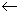is :Base classDerived Class.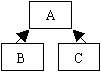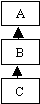(A)                                                         (B)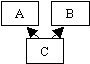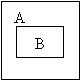(C)                                                      (D)

h.  What does the following line of code do?

TextField text = new TextField(10);

(A)    Creates 10 text objects.

(B)    Creates text object that can hold 10 characters.

(C)    Creates text object and initialize it with the value 10.

(D)    None of the above.

i.    What will be the output of the following code segment?

class A{

static int dummy(int i) {

if (i = = 1 || i = = 0)    return 1;

else   return (i * dummy(i - 1));

}

public static void main(String args[]) {

int      sum = 0;

for (int i = 6; i >= 0; i -= 2)

sum += dummy(i);

System.out.println(" Sum = " + sum);

}

}

(A)   746                                              (B)  874

(C) 747                                               (D)  875

j.    Given the code

String   s1 = "test";

String   s2 = new String(s1);

String   s3 = s1;

Which of the following would not equate to true?

(A)  s1 = = s2                                     (B) s1.equals(s2);

(C)  s3 = = s1                                     (D) s3.equals(s2)

Answer any FIVE Questions out of EIGHT Questions.

Each question carries 16 marks.

Q.2     a.   What is ARP? What is it used for?                                                                     (3)

b.   List the major features of the service provided by TCP? Briefly explain how congestion control is done in TCP?                                                  (8)

c.   Briefly explain specifying the protocol(s) involved, how does the traceroute program work?                                                                       (5)

Q.3     a.   How is fragmentation and reassembly of datagrams handled in IPv6? How does it differ from IPv4?                                                                       (8)

b.   Give the format of UDP datagram and explain the semantics of every field. How is the checksum in the header computed?                               (8)

Q.4     a.   What is MIME? What are the advantages of MIME over older e-mail systems?                 (5)

b.   What are socket APIs? What are they used for?                                                 (5)

c.   What do you understand by client-server paradigm? What are the main characteristics of the client and server software?                                  (6)

Q.5     a.   What is VoIP? What are its advantages over PSTN?                                          (4)

b.   Describe the Network File System (NFS) for remote file access. What are its advantages over FTP?                                                                    (5)

c.   Illustrate and explain how an active document gets converted from source form to the executable form.                                                                  (7)

Q.6     a.   Design an applet “Clicker” that displays a button and a text field on the screen. The text field must display the number of times the button is pressed.                                                                (8)

b.   Write the HTML code that displays “Click Count” in the title bar and executes the “Clicker” applet of Q.6 a.                                                           (4)

c.   What is reusability of code? Which features of object oriented paradigm help in code reusability and how?                                                             (4)

Q.7     a.   Write a Java program to count the number of lines in a file and then append “x” at the end of the file. Here x is the total number of lines in the file.                                                                   (8)

b.   What do you understand by CLASSPATH? Suppose the .class files are stored in a package MyPackage, in the package hierarchy: C19.JavaPrograms.MyPackage. Define the CLASSPATH for this. (4)

c.   What happens to the objects that are not in use by the program? Briefly explain, how Java manages such objects?                                              (4)

Q.8     a.   Define the necessary classes in Java for the following details: ‘Country’ is a super class with a data member: name. ‘Country’ has a sub class ‘State’ with data members: name and population. Write constructors for every class to initialize the data members. In ‘State’, define a member function: display() to display the output as: ‘State name’ is a state  in ‘Country name’.                                                                    (8)

b.   What is a Java Bean? Briefly explain the steps to create a new Bean.                  (8)

Q.9     a.   What do you understand by scope and lifetime of variables in Java? Explain.                       (4)

b.   What do you understand by multithreading? What facilities are provided by Java to handle concurrent access of a common data structure by two or more threads?                                                         (6)

c.   Write a program in Java Swing to display the picture of a tree stored in the file “tree.gif” and display the label as “Tree”.                                            (6)